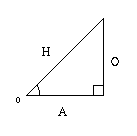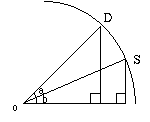[ / main / writing / 3d_tutorial ]

# Entering the 3rd Dimension©1996 Jeff Weeks and Codex softwareFirst of all, I'm probably a terrible teacher so if you don't understand this help file, don't worry. Check up on some more tutorials and hopefully they will describe the 3D process better than I do. I am not a professional and therefore may have some wrong information. I'm am, basically, just describing how I got 3D to work for me.Second of all, I took the time to write this little tutorial so I would expect a little bit of credit if you find this useful. This, and the source code, is copyrighted by me and Codex software. I will allow reproduction of this document as long as the origional copyright stays intact. Other than that, have fun and don't rip me off!If your looking for a really good tutorial on 3D check out the documentation for the Zed 3D engine. That's where I got a lot of my information from. The VLA and Asphixia demo trainers are also good sources of information. Unfortunately, I don't have URLs for any of the above :(Let's skip all the introductions and get right down and dirty. A basic 3D engine consists of three abilities:

. rotation
. translation
. projection## RotationNow, before I go to describe rotation, let me first do a quick intro to trigonometry. Trig. is basically the relations of a right angle triangle. The only functions you need to be aware of are sine and cosine: Your basic right angle triangle describes the following:

sine = (O/H)
cosine = (A/H)Those who already know trig. will notice that I am using angle o to calculate these ratios. This is the only angle you really need to be concerned with in 3D rotation. You should also note the O is the opposite side of angle o and A is the adjacent side of angle o. These are just common trigonometry terms.Now, consider the situation at left. You want to rotate the point S (source) to point D (destination). You can do this simply doing the following:

D.x = cos(a+b) * r
D.y = sin(a+b) * r

r is both the radius of the circle used to rotate, as well as both triangle's hypoteneuse'. That is probably important to note. Since we rotate around in a spherical system, the hypoteneuse never changes. Just as the radius of a circle never changes.Now, simple trigonometry dictates that:

sin(a+b) = sin(a) * cos(b) + cos(a) * sin(b)
cos(a+b) = cos(a) * cos(b) - sin(a) * sin(b)So we make the following substitutions to our origional equations to create the following equations:

D.x = r * cos(a) * cos(b) - r * sin(a) * sin(b)
D.y = r * sin(a) * cos(b) + r * cos(a) * sin(b)However, using a little sense, you can see that the following is true:

r * sin(a) = y
r * cos(a) = xJust think about it. The equation r * sin(a) is equivalent to H * (O/H). The H's cancel each other out and we're left with the O (opposite) side of a triangle. Which, incidentally, is our y coodinate. In the same way r * cos(a) is equivalent to H * (A/H). The H's cancel out and we're left with the A (adjacent) side of out triangle. This is our x coordinate. If this doesn't make since take a look at our origional trigonometry functions and triangle diagram.Now we finally get the below equations:

D.x = x * cos(b) - y * sin(b)
D.y = y * cos(b) + x * sin(b)Now, this above equation is actually only a 1D rotation though. How do we incorporate this into a 3D engine? Well, quite simply, by applying this 1D rotation three times! We just use the above equation and replace b for each of our X, Y and Z rotation values.It is important to realise that you must store your rotated object and the origional object in different locations. You do not want to overwrite the origional 3D object because it is used in this equation. It is your S (source) value, or x and y in the above equation.Here's some pseudo-code to put it all into perspective:

rotated.x = orig.x;
rotated.y = cos(x)*orig.y - sin(x)*orig.z;
rotated.z = sin(x)*orig.y + cos(x)*orig.z;
rotated.x = cos(y)*rotated.x - sin(y)*rotated.y;
rotated.y = sin(y)*rotated.x + cos(y)*rotated.y;
rotated.z = rotated.z;
rotated.x = cos(z)*rotated.x - sin(z)*rotated.z;
rotated.y = rotated.y;
rotated.z = sin(z)*rotated.x + cos(z)*rotated.z;You should note that in almost all languages, the sin and cos functions accept radians, not degrees, so it is necessary to convert them. This is done very easily using the following equation:

radian = degree * PI / 180## TranslationTranslation is the process of moving your 3D object in the direction of the x,y or z axis. This is done, very simply, by adding values to your rotated x,y and z values. It's really that simple:

translated_x = rotated_x + translation_value;
translated_y = rotated_y + translation_value;
translated_z = rotated_z + translation_value;Ofcourse, the translation values do not have to be, and rarely are, the same for x, y and z. The main reason for translation is to move your 3D object into place. The above 3D equations assume that your object is oriented with it's center at (0,0,0). Therefore you must move your 3D object from (0,0,0) to where it is to be placed.## ProjectionBut now that we've rotated an object, how do we display it on a 2D screen? This is where projection comes in. The simplest way of projecting a 3D object is to simply divide your rotated x and y values by your rotated z value. Then plotting these x and y values:

screen_x = x / z;
screen_y = y / z;However, there's one more thing. You must take into account the field of view. You do this simply by multiplying your x and y values before dividing by your z value. What you multiply by is determined by your screen size, and the field of view you want. Let's say you want a 60o field of view, and your screen size is 640x480. To calculate your multiplying variable (commonly called 'd' for 'distance of the projection plane') for your x variable you would do the following:

d=(screen_width/2) / tan(FOV/2)
d=320 / tan30
d=554The process is similar for the y value, you just replace screen_width, with screen_height. Now, the projection equation looks like this:

screen_x = (dx * x) / z;
screen_y = (dy * y) / z;However, we're still not done yet. The above projection equation assumes (0,0) is the top left corner of the screen. We don't want that, ofcourse, because we want (0,0) at the center of the screen. To correct this, simply add half the screen width to x, and half the screen height to y. The final projection equation looks like this:

screen_x = (dx * x) / z + (screen_width / 2);
screen_y = (dy * y) / z + (screen_height / 2);I hope that helped someone out.